# How To Read a Forex Currency Quote

forex trading is the buying of one currency for another. In other words it is the simultaneous buying of one currency and selling of another. In order to trade Forex, a forex trader must learn how to read a Forex quote. A forex currency quote is marked in terms of decimals - example forex trading quote of EURUSD: 1.2345

A forex currency quote represents the exchange rate between two currencies; referred to as forex currency pairs. Currencies in the forex market are traded in pairs, for example EURUSD, GBPUSD, USDCHF and USDJPY. The first one currency in the currency pair is known as the Base currency while the second currency in the currency pair is known as the Quote currency.

## Example of EURUSD Currency Pair - Forex Base Currency and Forex Quote Currency

EURUSD is one of the major currency pair in forex trading, therefore we shall use it for our example:

EURUSD

• Base Currency - EUR

This is the first currency in the currency pair (EUR). The Base currency is equal to 1 unit of the first currency.

• Quote Currency - USD

This is the second currency in the currency pair (USD). The Quote currency shows the value of the base currency in relation to the second currency.

If EURUSD currency pair forex quote = 1.4500

It means the ratio of EUR : USD = 1 : 1.4500

Therefore it means 1 Euro = 1.4500 US dollars

Example of exchange rates on MetaTrader 4 Forex Trading Platform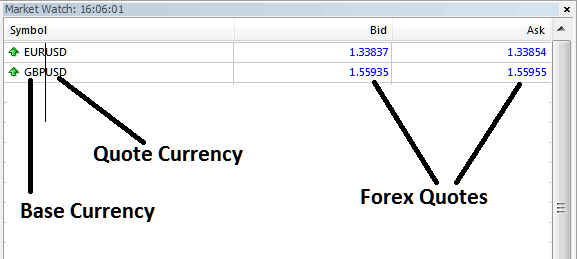Market Watch Window on MetaTrader 4 - MT4 Forex Symbols and Forex Currency Quotes

The above are examples forex quotes of EURUSD and GBPUSD currency pairs.

The Forex Quotes are shown as above, there is the Bid price, the price at which you buy and there is Ask price, the price at which you sell. The difference between these two Bid - Ask quotes is the spread. Spread is the small markup that the forex trading broker puts before selling to you. This forex spread is how forex brokers make profit.

## Where to Get Forex Quotes From MetaTrader 4 Forex Platform

To get the forex currency quotes display window on the MetaTrader 4 forex trading platform, follow these instructions, Click "View" Menu as shown below, then click "Market Watch" window.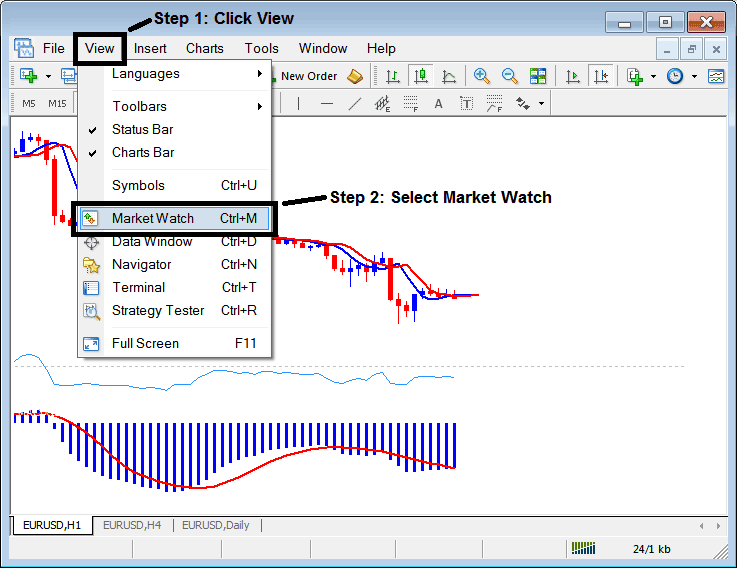Forex Currency Quotes and Forex MT4 Symbols for Forex Currency Pairs

The MT4 Market Watch window will display all the forex quotes of the various forex currency pairs as shown below.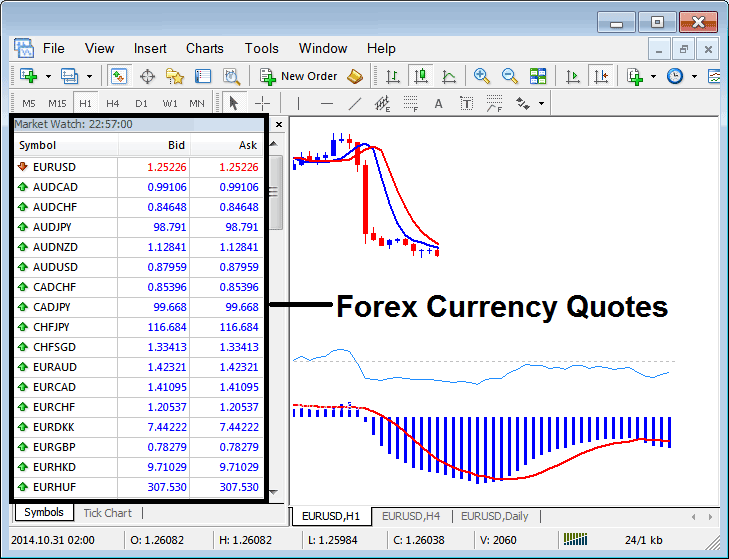List of Currency Quotes Displayed on MetaTrader 4 - MT4 Forex Currency Quotes - Forex Trading Quotes Explained## Pips - Forex Pips

One forex pip is the basic unit used to measure forex price movement; it is equal to the last decimal point in a Forex Currency Quote (4 Decimal Point Format), forex pip is the smallest change in forex trading price movement. The last decimal point should be the 4th decimal point. However, the fifth decimal point that is now implemented by online forex brokers is known as fraction of a pip or pipette.

## 5 Decimal Fractions Forex Quotes - Fraction of a Pip

From 2010, Forex Brokers started to implement the fifth decimal forex currency quotes, the fifth decimal point is known as the fractional Pip. Before this all forex quotes were 4 decimal places and that is when the last point was referred to as a pip. Therefore, when forex trading, as a trader always use the fourth decimal point and not the fifth decimal point as the pip value.

If you trade with a forex broker using 5 points (all forex trading brokers now use 5 decimal point currency quote format), then 1 fractional pip which is the 5th decimal place, will be equal to 1 dollar per 1 standard contract. However, this is just the Fraction of a pip and not the original Forex Pip. To Make up one Point (1 Forex Pip) you will have to use 10 points, (it is like rounding off to the fourth decimal point).

### Example Forex Currency Quote: For 4 Decimal Points Forex Quotes Format

This is how the forex quotes were displayed before using - 4 decimal points format

If EURUSD currency pair moves from 1.4500 to 1.4501 then this move is equal to:

1.4501-1.4500 = 0.0001 (1 forex pip)

Value of = 0.0001 USD

1 forex contract = \$100,000

Value of 1 pip for 1 forex contract = 100,000* 0.0001= \$10

1 forex pip = \$10, for 4 Decimal Points Forex Currency Quote Format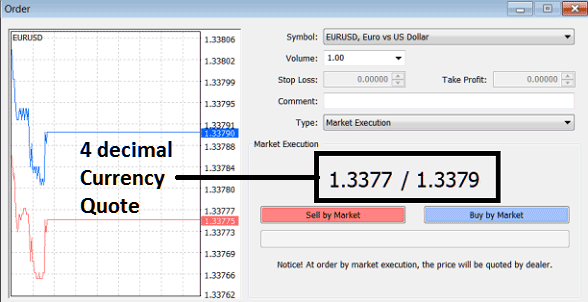4 Points Decimal Format on MetaTrader 4 - 4 Decimal Points Forex Currency Quote Format - How to Interpret Forex Currency Quotes

### Example Forex Currency Quote: For 5 Decimal Points Forex Quote Format

If EURUSD currency pair moves from 1.45000 to 1.45001 then this move is equal to:

1.45001-1.45000 = 0.00001 (1/10 pipette)

Value of = 0.00001 USD

1 forex contract = \$100,000

Value of 1/10 pipette for 1 forex contract = 100,000* 0.00001= \$1

1/10 forex pipette (Fraction of a Pip) = \$1, for 5 Decimal Points Forex Currency Quote Format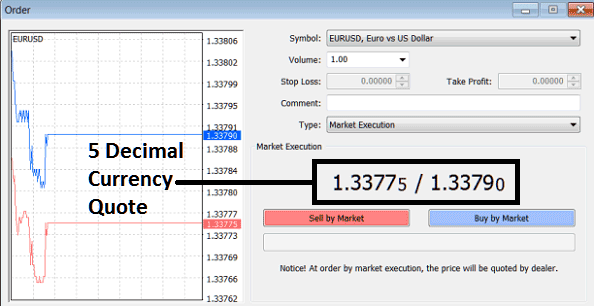5 Decimal Points Format on MetaTrader 4 - 5 Decimal Points Forex Currency Quote Format - How to Read Forex Currency Quotes

Please Note from the above MT4 forex platform screenshot how the fifth decimal point is shown in a digit much smaller than the other digits, this is to signify that it is a fraction of a forex pip.

## Interpreting Forex Currency Quotes Moves With Forex Charts

These streaming forex currency quotes in Forex are used to plot and draw forex trading charts - these forex charts are then used to analyze the forex chart price movements. When a Forex Trading Chart is plotted and drawn, forex traders can then use these forex charts to analyze the forex price movements, for example the forex price may show a downward forex price move or an upward forex price move. These forex price moves may be referred to as forex trends and the forex trend may be upward or downward - based on the direction of the forex prices - upward forex trend is when forex prices generally move upwards - downward forex trend is when forex prices generally move downwards.

Therefore as a beginner forex day trader all you need to know is that the forex currency quotes moves will be analyzed from these forex trading charts.